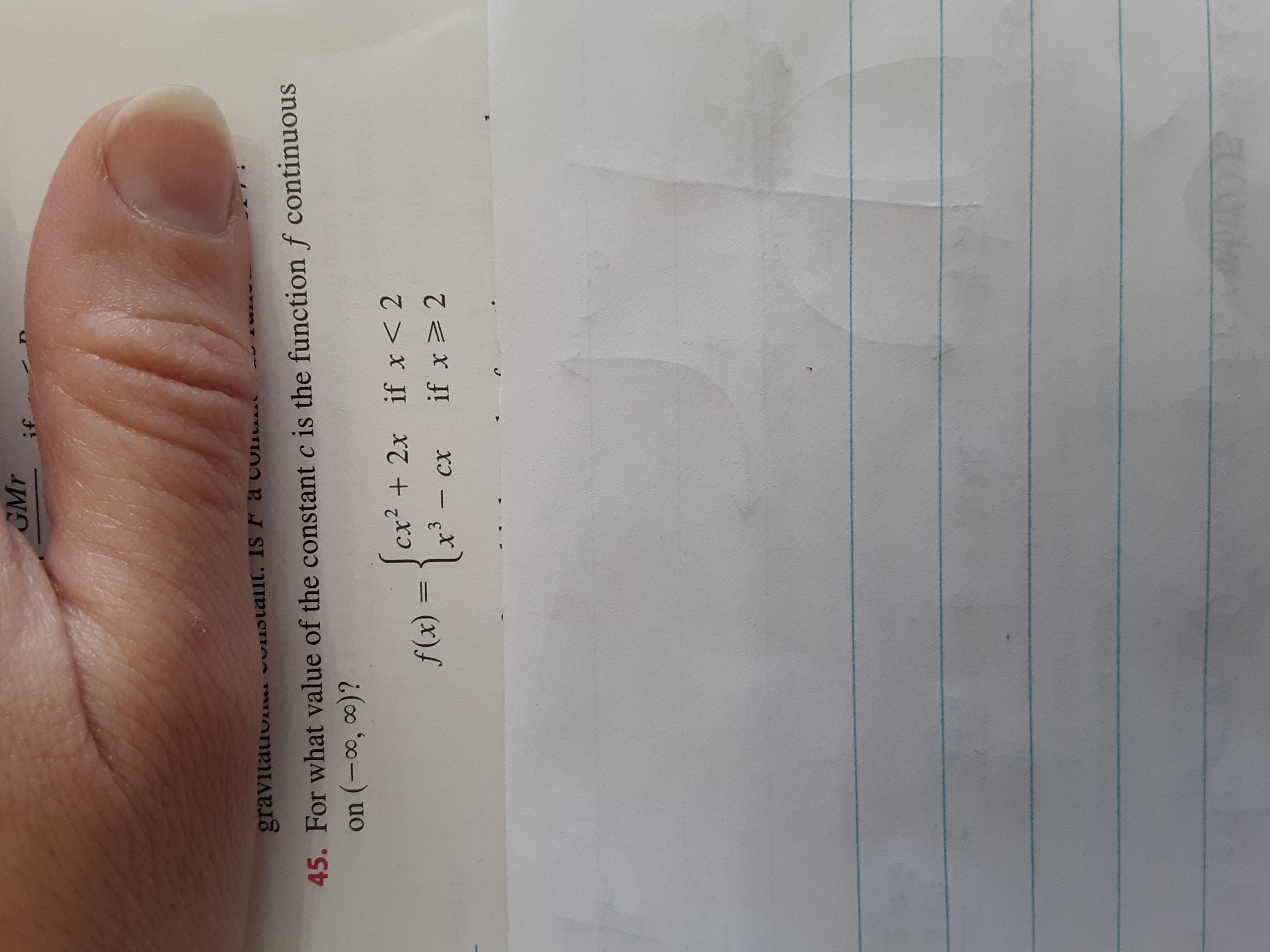# GMrifgravitauvivslallt. Is F a colit.45. For what value of the constant c is the function f continuouson (-0o, 0)?cx? + 2x if x< 2f(x) =1. - cxif x> 2

Question
2 views

See picture below.

Can you please show me the steps to solve this?help_outlineImage TranscriptioncloseGMr if gravitauvi vslallt. Is F a colit. 45. For what value of the constant c is the function f continuous on (-0o, 0)? cx? + 2x if x< 2 f(x) = 1. - cx if x> 2 fullscreen
check_circle

Step 1

The given function is

Step 2

To find: The value of (c) for which the given function is continuous.

Step 3

For the given function to be continuous the limit of function f(x) at x=2 from left an...

### Want to see the full answer?

See Solution

#### Want to see this answer and more?

Solutions are written by subject experts who are available 24/7. Questions are typically answered within 1 hour.*

See Solution
*Response times may vary by subject and question.
Tagged in

### Continuity